# Computer Science : Common Data Structures

## Example Questions

### Example Question #1 : Strings

Which of the following blocks of code converts an array of characters into a string?

private static void string() {

char[] vals = {'A','t', ' ', '6',' ','a','m','!'};

String s;

for(int i = 0; i < vals.length; i++) {

s += vals[i];

}

}

private static void string() {

char[] vals = {'A','t', ' ', '6',' ','a','m','!'};

String s = "";

for(int i = 0; i < vals.length; i++) {

vals[i] = s;

}

}

private static void string() {

char[] vals = {'A','t', ' ', '6',' ','a','m','!'};

String s = "";

for(int i = 0; i < vals.length; i++) {

s = vals[i];

}

}

private static void string() {

char[] vals = {'A','t', ' ', '6',' ','a','m','!'};

String s = "";

for(int i = 0; i < vals.length; i++) {

s += vals[i];

}

}

private static void string() {

String s = "At 6 am!";

char[] vals = new char[s.length()];

for(int i = 0; i < s.length(); i++) {

vals[i] = s.charAt(i);

}

}

private static void string() {

char[] vals = {'A','t', ' ', '6',' ','a','m','!'};

String s = "";

for(int i = 0; i < vals.length; i++) {

s += vals[i];

}

}

Explanation:

The easiest way to consider this is by commenting on the correct answer.  You must begin by defining the character array:

char[] vals = {'A','t', ' ', '6',' ','a','m','!'};

Next, you must initialize the string value s to be an empty string.  This is critical.  Otherwise, you can't build your string!

String s = "";

Next, you have the loop.  This goes through the characters and concatenates the values to the variable s. The operation to concatenate the characters is the "+=". This will give you the string value of the array of characters.

### Example Question #1 : Strings

See code below:

String[] books = {

"De Secundis Intentionibus",

"Leviathan",

"Averrois Commentaria Magna in Aristotelem De celo et mundo",

"Logica Docens for Idiots",

"Logica Utens for Tuba Players"

};

String userInput;

// In code excised from here, a person inputs the value "Logica Utens for Tuba Players" ...

for(int i = 0; i < books.length; i++) {

if(books[i] == userInput) {

System.out.println("This is a wonderful book!!");

}

}

What is the error in the code above?

The string comparison.

The use of the [] operator on the array.

It overruns an array.

The way the array is declared.

The use of if on an array element.

The string comparison.

Explanation:

The only major issue with this code is the use of the == operator to compare the two strings.  Since the user has input this value, will not have an equality on this test.  You must use the method .equals in order to check whether two strings are equal.  The code should be:

if(books[i].equals(userInput)){

...

(There are some cases in which == will work for string comparison, namely when literals are involved.  However, you should avoid relying on this and always use .equals().)

### Example Question #1 : Common Data Structures

String[] books = {

"Logica sive Ars Rationalis",

"Kritik der reinen Vernunft",

"Cursus Philosophicus Thomisticus",

"Happy words for happy people",

"Insane words for an insane world"

};

String str = "Kittens in a cart";

ArrayList<String> vals = new ArrayList<String>();

for(int i = 0; i < books.length; i++) {

if(books[i].compareTo(str) > 0) {

}

}

System.out.println(vals);

What is the output for this method?

[]

[Cursus Philosophicus Thomisticus, Happy words for happy people, Insane words for an insane world]

[Kritik der reinen Vernunft, Logica sive Ars Rationalis]

[Happy words for happy people, Insane words for an insane world]

[Logica sive Ars Rationalis, Kritik der reinen Vernunft]

[Logica sive Ars Rationalis, Kritik der reinen Vernunft]

Explanation:

The compareTo method for strings compares to string objects and returns:

• 0 when they are equal
• Something positive when the given string is alphabetically (really, lexicographically) later than the argument to the method.
• Something negative when the given string is alphabetically (really, lexicographically) before the argument to the method.

Our strings could be put in this order:

"Cursus Philosophicus Thomisticus"

"Happy words for happy people"

"Insane words for an insane world"

"Kritik der reinen Vernunft"

"Logica sive Ars Rationalis"

For each of these, we are asking, "Is it later in order than 'Kittens in a cart'?"  This is true for "Kritik der reinen Vernunft" and "Logica sive Ars Rationalis".  Thus, our output is:

[Logica sive Ars Rationalis, Kritik der reinen Vernunft]

Notice the order—this is due to the order in the original array.

### Example Question #1 : Strings

String greet = "Hello ";
String sub;
int len = greet.length();
sub = greet.substring(0, (len/2));
System.out.println (sub);

What is printed?

Hel

Hell

Hello

He

Error

Hel

Explanation:

The length of greet is 6 characters including the space at the end.

greet.substring(0, (len/2)) is equal to greet.substring(0, 3)

The substring of greet from the zeroth position to second position, not to the third position.

### Example Question #1 : Trees

On a standard binary tree, what would the data structure look like if we inserted the following names into the tree, supposing that names are compared in a standard lexicographic order:

Isaac, Henrietta, Nigel, Buford, Jethro, Cletus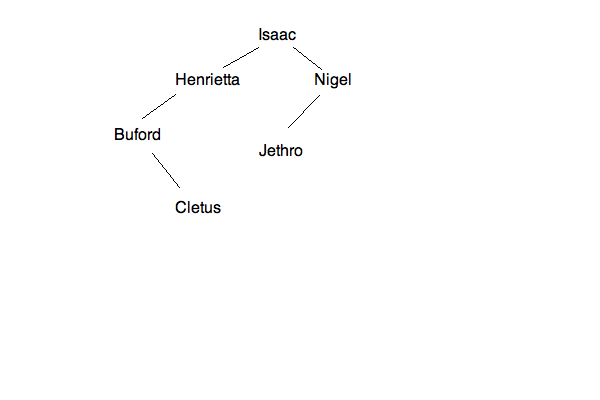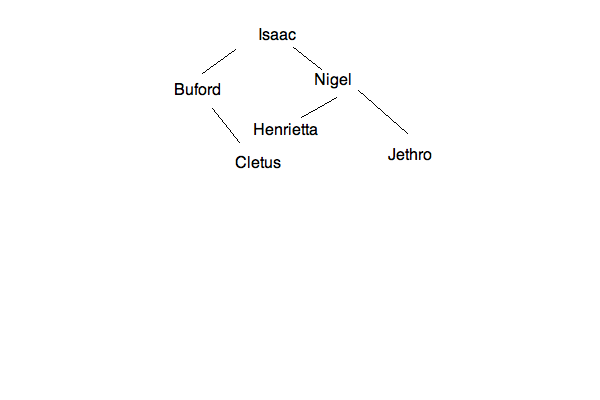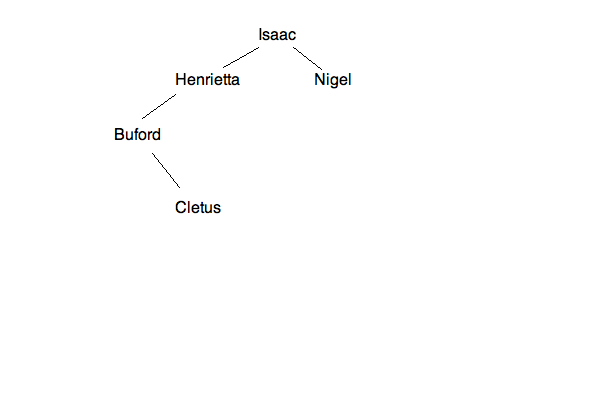None of the trees are correct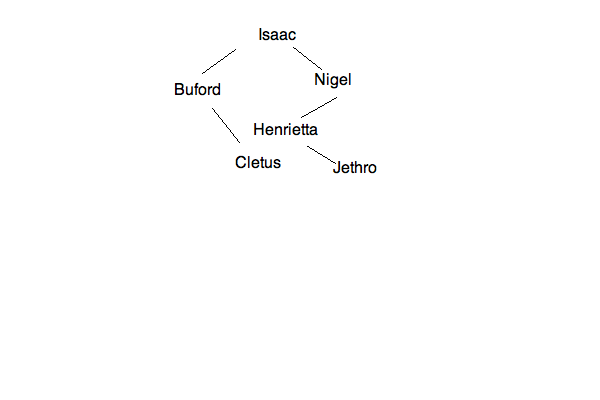Explanation:

A standard Binary Tree inserts into the root first.  It then tries to insert to the "left" for values that are smaller and to the "right" for values that are larger.  Therefore, for the data given, we have the first step: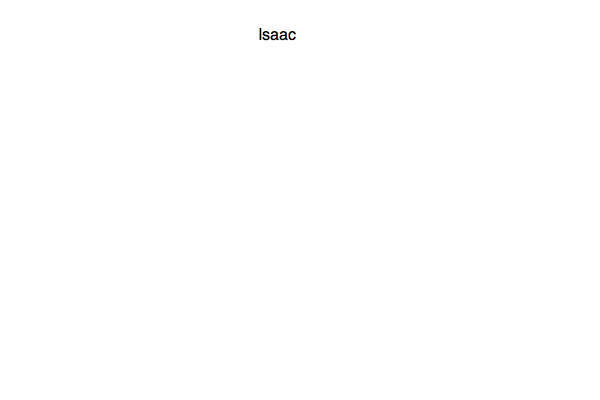Next, you will insert "Henrietta" to the left, for that is less than "Isaac":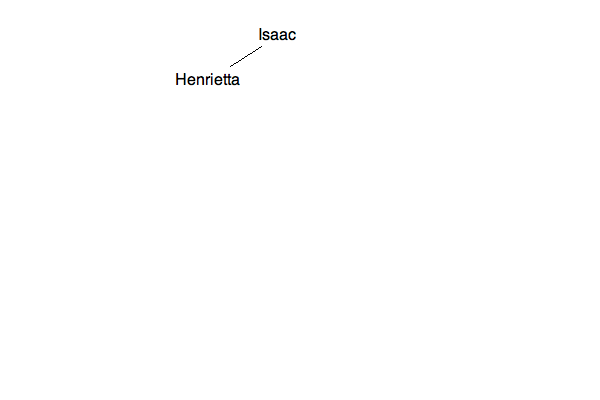Next, "Nigel" is greater than "Isaac":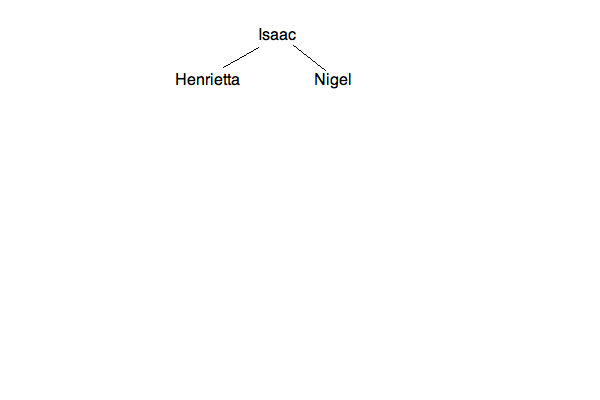Then, "Buford" is less than "Isaac" and then less than "Henrietta":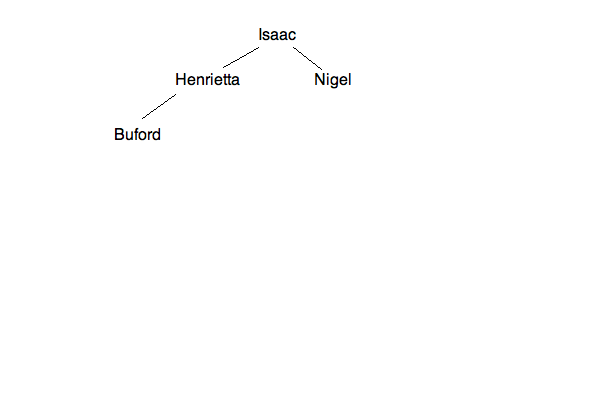This continues through the following stages: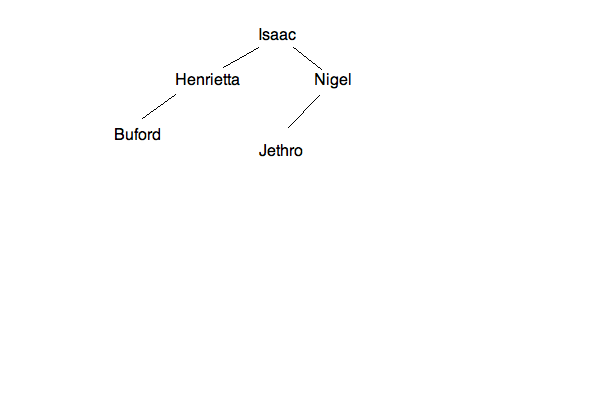Thus, the last image is your final tree!

## POST-ORDER TRAVERSAL

GIVEN THE FOLLOWING TREE: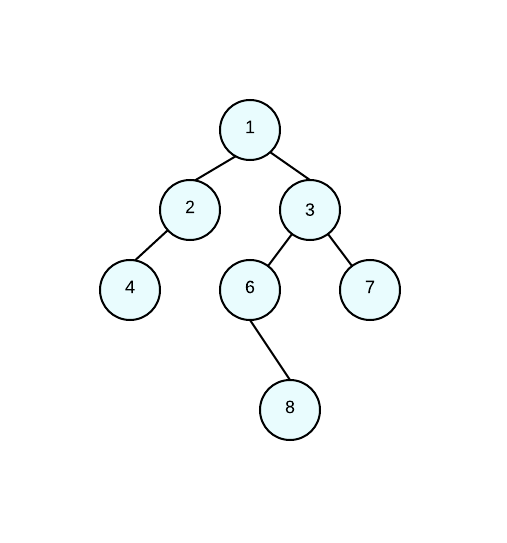WHAT IS THE RESULT OF A POST ORDER TRAVERSAL?

POST ORDER TRAVERSAL: 8, 7, 6, 4, 3, 2, 1

POST ORDER TRAVERSAL: 1, 2, 3, 4, 6, 7, 8

POST ORDER TRAVERSAL: 1, 2, 3, 4, 6, 7, 8

POST ORDER TRAVERSAL: 4, 2, 8, 6, 7, 3, 1

POST ORDER TRAVERSAL: 4, 2, 8, 6, 7, 3, 1

Explanation:

When doing a post-order traversal we go in the following order:

left, right, root.

This means that we are doing any left nodes first, then the right nodes, and LASTLY, the root nodes. If a node is a parent, then we must go throught the left and right children first. Since we're doing post-order traversal, the main root is going to be LAST.

In our example, 1 is a parent so we go to it's left child who is also a parent to node 4. This means that 4 is our first number in the traversal.

POST ORDER TRAVERSAL: 4

Since node 2 doesn't have a right child, we then make that node our next number in the traversal since node 2 it's the left child of node 1.

POST ORDER TRAVERSAL: 4, 2

By now, we have traversed the left nodes of the root. Now we move on to the right subtrees of node 1. Since node 3 is a parent node, we go to it's left child first (node 6). Since node 6 is also a parent, we move on to its children. Node 6 doesn't have a left child. Therefore our next number in the traversal is its right child (node 8) and then the subtree's root (node 6).

POST ORDER TRAVERSAL: 4, 2, 8, 6

Now, we've traversed the left children of node 3 we need to traverse the right child (node 7) who doesn't have any children of its own.

POST ORDER TRAVERSAL: 4, 2, 8, 6, 7

Lastly since we traversed it's right child, we move to the parent and traverse node 3 and our main root (node 1).

POST ORDER TRAVERSAL: 4, 2, 8, 6, 7, 3, 1

### Example Question #1 : Lists

Consider the code below:

ArrayList<String> myPhilosophers = new ArrayList<String>();

for(int i = 0; i < myPhilosophers.size(); i++) {

String s = myPhilosophers.get(i);

if(s.charAt(0) >= 'H' || s.charAt(3) < 'd') {

myPhilosophers.set(i, s.toUpperCase());

}

}

System.out.println(myPhilosophers);

What is the output for the code above?

[FREGE, HUSSERL, HEGEL, BILL, FREDERICK]

[Frege, HUSSERL, HEGEL, Bill, FREDERICK]

[Frege, HUSSERL, HEGEL, Bill, Frederick]

[FREGE, Husserl, Hegel, Bill, FREDERICK]

[Frege, Husserl, Hegel, Bill, FREDERICK]

[Frege, HUSSERL, HEGEL, Bill, Frederick]

Explanation:

Consider the logic that is in the loop.  The if statement will be reached either if you:

1. Have a first character that is H or later in the alphabet (in capital letters).
2. Have a fourth character that is less than d

The first case applies to "Husserl" and "Hegel."  However, the second does not apply to any—not even to "Frederick", for d is equal  to d, not less than it!

### Example Question #1 : Lists

Consider the code below:

ArrayList<String> names = new ArrayList<String>();

for(int i = 0; i < 12; i++) {

for(int i = 0; i < 12; i++) {

if(names.get(i).equals("Frege")) {

names.remove(i);

}

}

What is the bug in the code above?

The list will overrun its indices in the second loop.

There are no bugs.

The .get method is buggy and likely to crash.  It is better to use an Iterator.

The list will use up all of the memory on the machine.

There is an infinite loop in one of the two loops.

The list will overrun its indices in the second loop.

Explanation:

In the second loop, when you remove one of the items, you thus make the ArrayList one element shorter.  This means that if you attempt to go through to index 11, you will receive an error at some point, for the list will have "shrunk".  This is like an array out of bounds error, though it will be an IndexOutOfBoundsException exception.

### Example Question #1 : Lists

Consider the following code:

public static class Circle {

public Circle(double r) {

}

public double getArea() {

}

}

public static void main(String[] args) {

ArrayList<Circle> circles = new ArrayList<Circle>();

for(int i = 0; i < 10; i++) {

circles.add(new Circle(i + 4 * 2));

}

}

Which of the following represents code for iterating through the list circles in order to output the areas of the circles contained therein?

for(int i = 0; i < circles.size; i++) {

System.out.println(circles.get()[i].getArea());

}

for(int i = 0; i < circles.size(); i++) {

System.out.println(circles.get(i).getArea());

}

for(int i = 0; i < circles.size(); i++) {

System.out.println(circles[i].getArea());

}

for(int i = 0; i < circles.size(); i++) {

System.out.println(circles[i].get().getArea());

}

for(int i = 0; i < circles.length; i++) {

System.out.println(circles[i].getArea());

}

for(int i = 0; i < circles.size(); i++) {

System.out.println(circles.get(i).getArea());

}

Explanation:

In the answers, there are only two issues to consider.  On the one hand, look at the loop control statement.  It is some variant on:

circle.size()

and

circles.length

For the List types, you need to use the method size()not length (which you use for arrays).  Likewise, one way to extract elements from the ArrayList type is to use the .get method.  You must use this and not the brackets [] that you use for arrays.

### Example Question #1 : Lists

Which of the following is NOT a valid declaration for an ArrayList?

ArrayList list = new ArrayList();

List list = new ArrayList();

List list = new ArrayList();

ArrayList list = new ArrayList();

ArrayList list = new ArrayList();

ArrayList list = new ArrayList();